# WO2015029744A1 - Transformer device - Google Patents

## Info

Publication number
WO2015029744A1
WO2015029744A1 PCT/JP2014/070967 JP2014070967W WO2015029744A1 WO 2015029744 A1 WO2015029744 A1 WO 2015029744A1 JP 2014070967 W JP2014070967 W JP 2014070967W WO 2015029744 A1 WO2015029744 A1 WO 2015029744A1
Authority
WO
WIPO (PCT)
Prior art keywords
transformer
circuit
line
reactance
lines
Prior art date
Application number
PCT/JP2014/070967
Other languages
French (fr)
Japanese (ja)
Inventor

Original Assignee

Priority date (The priority date is an assumption and is not a legal conclusion. Google has not performed a legal analysis and makes no representation as to the accuracy of the date listed.)
Filing date
Publication date
Priority to JP2013178494A priority Critical patent/JP5695712B2/en
Priority to JP2013-178494 priority
Application filed by 住友電気工業株式会社, 国立大学法人豊橋技術科学大学 filed Critical 住友電気工業株式会社
Publication of WO2015029744A1 publication Critical patent/WO2015029744A1/en

• 238000010586 diagrams Methods 0.000 description 43
• 230000001131 transforming Effects 0.000 description 15
• 239000003990 capacitor Substances 0.000 description 12
• 230000003071 parasitic Effects 0.000 description 3
• 229910002601 GaN Inorganic materials 0.000 description 2
• 230000001939 inductive effects Effects 0.000 description 2
• 229910003465 moissanite Inorganic materials 0.000 description 2
• 229910010271 silicon carbide Inorganic materials 0.000 description 2
• 281000076521 Chubu Electric Power companies 0.000 description 1
• 280000024908 Next Generation companies 0.000 description 1
• 230000005540 biological transmission Effects 0.000 description 1
• 230000015556 catabolic process Effects 0.000 description 1
• 230000001276 controlling effects Effects 0.000 description 1
• 230000000875 corresponding Effects 0.000 description 1
• 230000001808 coupling Effects 0.000 description 1
• 238000010168 coupling process Methods 0.000 description 1
• 238000005859 coupling reactions Methods 0.000 description 1
• 230000014509 gene expression Effects 0.000 description 1
• 230000004048 modification Effects 0.000 description 1
• 238000006011 modification reactions Methods 0.000 description 1
• 239000003921 oils Substances 0.000 description 1
• 239000004065 semiconductors Substances 0.000 description 1

## Images

•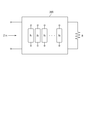•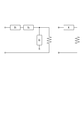•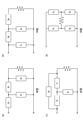•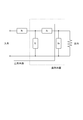•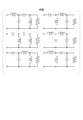•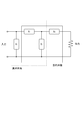•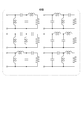•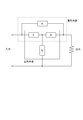•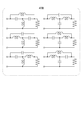•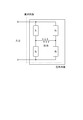•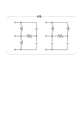•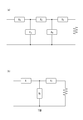•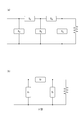•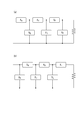•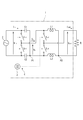•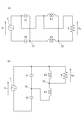•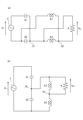•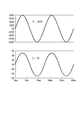•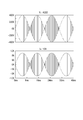•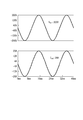••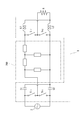•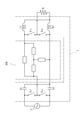•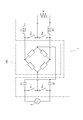## Classifications

• HELECTRICITY
• H02GENERATION; CONVERSION OR DISTRIBUTION OF ELECTRIC POWER
• H02MAPPARATUS FOR CONVERSION BETWEEN AC AND AC, BETWEEN AC AND DC, OR BETWEEN DC AND DC, AND FOR USE WITH MAINS OR SIMILAR POWER SUPPLY SYSTEMS; CONVERSION OF DC OR AC INPUT POWER INTO SURGE OUTPUT POWER; CONTROL OR REGULATION THEREOF
• H02M5/00Conversion of ac power input into ac power output, e.g. for change of voltage, for change of frequency, for change of number of phases
• H02M5/02Conversion of ac power input into ac power output, e.g. for change of voltage, for change of frequency, for change of number of phases without intermediate conversion into dc
• H02M5/04Conversion of ac power input into ac power output, e.g. for change of voltage, for change of frequency, for change of number of phases without intermediate conversion into dc by static converters
• H02M5/06Conversion of ac power input into ac power output, e.g. for change of voltage, for change of frequency, for change of number of phases without intermediate conversion into dc by static converters using impedances
• HELECTRICITY
• H02GENERATION; CONVERSION OR DISTRIBUTION OF ELECTRIC POWER
• H02MAPPARATUS FOR CONVERSION BETWEEN AC AND AC, BETWEEN AC AND DC, OR BETWEEN DC AND DC, AND FOR USE WITH MAINS OR SIMILAR POWER SUPPLY SYSTEMS; CONVERSION OF DC OR AC INPUT POWER INTO SURGE OUTPUT POWER; CONTROL OR REGULATION THEREOF
• H02M5/00Conversion of ac power input into ac power output, e.g. for change of voltage, for change of frequency, for change of number of phases
• H02M5/02Conversion of ac power input into ac power output, e.g. for change of voltage, for change of frequency, for change of number of phases without intermediate conversion into dc
• H02M5/04Conversion of ac power input into ac power output, e.g. for change of voltage, for change of frequency, for change of number of phases without intermediate conversion into dc by static converters
• H02M5/22Conversion of ac power input into ac power output, e.g. for change of voltage, for change of frequency, for change of number of phases without intermediate conversion into dc by static converters using discharge tubes with control electrode or semiconductor devices with control electrode
• H02M5/275Conversion of ac power input into ac power output, e.g. for change of voltage, for change of frequency, for change of number of phases without intermediate conversion into dc by static converters using discharge tubes with control electrode or semiconductor devices with control electrode using devices of a triode or transistor type requiring continuous application of a control signal
• H02M5/293Conversion of ac power input into ac power output, e.g. for change of voltage, for change of frequency, for change of number of phases without intermediate conversion into dc by static converters using discharge tubes with control electrode or semiconductor devices with control electrode using devices of a triode or transistor type requiring continuous application of a control signal using semiconductor devices only
• HELECTRICITY
• H03BASIC ELECTRONIC CIRCUITRY
• H03HIMPEDANCE NETWORKS, e.g. RESONANT CIRCUITS; RESONATORS
• H03H7/00Multiple-port networks comprising only passive electrical elements as network components
• H03H7/38Impedance-matching networks

## Abstract

Provided is a transformer device provided between a power source and a load with a resistance value R, wherein, where n is a natural number of at least 4, provided is a two terminal pair circuit configured by mutually connecting n reactance elements, and with respect to a discretionary resistance value R of the load, a real number component of an input impedance Zin of the two terminal pair circuit is represented by k·R, where k is a constant, and an imaginary component is 0. This manner of transformer device is small and light, and does not require the coil, iron core or the like of conventional transformers.

## Description

Transformer

The present invention relates to a transformer device.

Transformers are used for commercial AC transmission and distribution systems. Near the customer, for example, a pole transformer that transforms 6600 V (50 Hz or 60 Hz) to 200 V is used (see Non-Patent Document 1). In such a transformer on a pole, a thick coil serving as a conducting wire is wound around an iron core and has a corresponding weight. Further, if insulating oil and a case are further included, for example, a type having a diameter of 40 cm and a height of 80 cm has a weight of about 200 kg.

On the other hand, research on SST (Solid-State Transformer) is being conducted for the realization of smart grid, which is the next generation power system. A high-frequency transformer is used for SST (see, for example, Non-Patent Document 2).

Chubu Electric Power website, "Pole-top transformer", [online], [Search on July 19, 2013], Internet <URL: http://www.chuden.co.jp/e-museum/guide/3floor/ exhibit_c23.html> Falcones, S .: et al., Power and Energy Society General Meeting, 2010 IEEE, pp. 1-8, Minneapolis, July 2010

Conventional pole transformers are heavy and are therefore not easy to handle. Also, a large mounting space is required on the column to accommodate the external dimensions.
On the other hand, the high-frequency transformer has a design difficulty because the influence of parasitic capacitance cannot be avoided.

In view of such conventional problems, the present invention provides a revolutionary next-generation transformer that is small and light and does not require magnetic coupling, electromagnetic induction, a coil for mutual inductance, an iron core, or the like as in a conventional transformer. The purpose is to provide.

The present invention relates to a transformer provided between a power supply and a load having a resistance value R, and a two-terminal pair circuit configured by connecting n reactance elements to each other, where n is a natural number of 4 or more. with respect to any of the resistance value R of the load, the input impedance Z in of the two-port network, the real component, the k as a constant, is represented by k · R, and in the imaginary component is zero There is a transformer.
The reactance element is an inductor having inductive reactance or a capacitor having capacitive reactance.

By using the transformer device of the present invention as a power transformer, a conventional transformer including a coil and an iron core becomes unnecessary. Therefore, a dramatic reduction in size and weight of the transformer and a cost reduction associated therewith can be realized.

It is a figure which shows the concept of the transformation apparatus by a two terminal pair circuit (four terminal circuit). It is a figure which shows the view of the number of elements which comprise a circuit. It is a figure which shows 4 patterns of the circuit structures which can be comprised by the minimum number of elements 4. FIG. It is a figure which shows 4A type circuit structure. It is a figure which shows the example 6 pattern of a 4A type circuit structure. It is a figure which shows 4B type circuit structure. It is a figure which shows the example 6 pattern of a 4B type circuit structure. It is a figure which shows 4C type circuit structure. It is a figure which shows the example 6 pattern of a 4C type circuit structure. It is a figure which shows 4D type circuit structure. It is a figure which shows the example 2 pattern of a 4D type circuit structure. (A) is a figure which shows the circuit structure of the 1st example of n = 5. (B) shows a T-type circuit. (A) is a figure which shows the circuit structure of the 2nd example of n = 5. (B) shows a π-type circuit. (A) is a figure which shows the circuit structure of the 1st example of n = 6. (B) is a figure which shows the circuit structure of the 2nd example of n = 6. It is a circuit diagram which shows the transformer by another structure. FIG. 16A is a circuit diagram illustrating a state of actual connection when two switches on the upper side of the four switches in FIG. 15 are on and two switches on the lower side are off. (B) is the circuit diagram which rewritten the same circuit diagram as (a) in step shape. FIG. 16A is a circuit diagram illustrating a state of actual connection when two switches on the lower side are on and two switches on the upper side are off among the four switches in FIG. 15. (B) is a circuit diagram in which the same circuit diagram as (a) is rewritten in a staircase pattern. It is a wave form diagram showing the input voltage and input current with respect to the transformer of FIG. FIG. 16 is a waveform diagram illustrating voltage and current in an intermediate stage of voltage transformation in the voltage transformer of FIG. 15. FIG. 16 is a waveform diagram illustrating an output voltage and an output current from the transformer device of FIG. 15. FIG. 16 is a circuit diagram in which the transformer device shown in FIG. 15 and the transformer device having a 4A type circuit configuration are combined. FIG. 16 is a circuit diagram in which the transformer device shown in FIG. 15 and the transformer device having a 4B type circuit configuration are combined. FIG. 16 is a circuit diagram in which the transformer device shown in FIG. 15 and the transformer device having a 4C type circuit configuration are combined. FIG. 16 is a circuit diagram in which the transformer device shown in FIG. 15 and the transformer device having a 4D type circuit configuration are combined.

[Summary of Embodiment]
The gist of the embodiment of the present invention includes at least the following.

(1) This is a transformer provided between a power source and a load having a resistance value R, and a two-terminal pair configured by connecting n reactance elements to each other, where n is a natural number of 4 or more. The input impedance Z in of the two-terminal pair circuit is expressed by k · R, where k is a constant, and the imaginary component is 0 is a transformer.
In the transformer apparatus as described above, an output voltage proportional to the input voltage can be obtained regardless of the resistance value R of the load. That is, a transformer device that converts an input voltage into an output voltage at a constant transformation ratio (1 / k) 1/2 is obtained. By using such a transformer as a transformer, a conventional commercial frequency transformer or a high-frequency transformer becomes unnecessary. Therefore, a dramatic reduction in size and weight of the transformer and a cost reduction associated therewith can be realized. Furthermore, the problems of parasitic capacitance and leakage magnetic field generation, which are problems with high-frequency transformers, are eliminated, and a low-loss transformer can be realized.

(2) Also, in the transformer of (1), assuming that the reactances of the four reactance elements are X 1 , X 2 , X 3 , and X 4 , respectively, in order from the input side, 1 of the two-terminal pair circuit X 2 is between X 1, 2-wire in the line, the said two-port network by X 4 in between X 3, 2-wire in the 1 line is constructed, it is preferable that the following conditions are met.
(1 / X 1 ) + (1 / X 2 ) + (1 / X 3 ) = 0 ∧ X 2 + X 3 + X 4 = 0
In this case, the input impedance Z in is Z in = (X 2 2 / X 4 2 ) · R, and is proportional to the resistance value R of the load, and an output voltage proportional to the input voltage is obtained.
The symbol “∧” represents a logical product (the same applies hereinafter).

(3) Also, in the transformer of (1), assuming that the reactances of the four reactance elements are X 1 , X 2 , X 3 , and X 4 , 2 in the two-terminal pair circuit in order from the input side. X 1, 1 on the line X 2, X 3 is in between the two wires in between the lines, wherein the two-port network by X 4 in 1 line is constructed, it is preferable that the following conditions are met.
X 1 + X 2 + X 3 = 0 (1 / X 2 ) + (1 / X 3 ) + (1 / X 4 ) = 0
In this case, the input impedance Z in is Z in = (X 1 2 / X 3 2 ) · R, which is proportional to the resistance value R of the load and an output voltage proportional to the input voltage is obtained.

(4) Also, in the transformer of (1), assuming that the reactances of the four reactance elements are X 1 , X 2 , X 3 , and X 4 , respectively, in the order of the two-terminal pair circuit as viewed from the input side. X 2 is between X 1, 2-wire in the line, the by the T-type circuit composed, and X 4 in parallel with the series of X 1 and X 3 by X 3, in the one line It is preferable that a two-terminal pair circuit is configured and the following conditions are satisfied.
X 1 + X 3 + X 4 = 0 (1 / X 1 ) + (1 / X 2 ) + (1 / X 3 ) = 0
In this case, the input impedance Z in is Z in = (X 1 2 / X 3 2 ) · R, which is proportional to the resistance value R of the load and an output voltage proportional to the input voltage is obtained.

(5) Also, in the transformer of (1), assuming that the reactances of the four reactance elements are X 1 , X 2 , X 3 , and X 4 , the two-terminal pair circuit 2 in order from the input side. A first series body of X 1 , X 2 between the lines and a second series body of X 3 , X 4 between the two lines, the interconnection point of the first series body and the second series body It is preferable that the two-terminal pair circuit in which the interconnection point is an output terminal is configured and the following conditions are satisfied.
X 1 + X 2 + X 3 + X 4 = 0 (1 / X 1 ) + (1 / X 2 ) + (1 / X 3 ) + (1 / X 4 ) = 0
In this case, the input impedance Z in is Z in = {(X 1 + X 2 ) 2 / (X 1 −X 2 ) 2 } · R, which is proportional to the resistance value R of the load and the output voltage proportional to the input voltage. Is obtained.

(6) Also, in the transformer of (1), when the reactances of the five reactance elements are X A , X B , X C , X D , and X E , the two terminal pairs are sequentially viewed from the input side. The two-terminal pair circuit is configured by X A on one line of the circuit, X B between the two lines, X C on the one line, X D between the two lines, and X E on the one line. ,
X A = -X B ∧ X E = -X D ∧ X C = X A + X E
It is preferable that the relationship is
In this case, the input impedance Z in is Z in = (X A 2 / X E 2 ) · R, which is proportional to the resistance value R of the load and an output voltage proportional to the input voltage is obtained.

(7) Also, in the transformer of (1), when the reactances of the five reactance elements are X A , X B , X C , X D , and X E , the two terminal pairs are sequentially viewed from the input side. The two-terminal pair circuit is configured by X A between two lines of the circuit, X B on one line, X C between two lines, X D on the one line, X E between the two lines. ,
X A = −X B ∧X E = −X D ＣX C = X A · X E / (X A + X E )
It is preferable that the relationship is
In this case, the input impedance Z in is Z in = (X A 2 / X E 2 ) · R, which is proportional to the resistance value R of the load and an output voltage proportional to the input voltage is obtained.

(8) Also, in the transformer of (1), when the reactances of the six reactance elements are X A , X B , X C , X D , X E , and X F , respectively, X A on one line of a two-terminal pair circuit, X B between two lines, X C on the one line, X D between the two lines, X E on the one line, X between the two lines F , to form the two-terminal pair circuit,
X A = X C = −X B ∧X D = X F = −X E
It is preferable that the relationship is
In this case, the input impedance Z in is Z in = (X A 2 / X F 2 ) · R, and an output voltage proportional to the resistance value R of the negative load and proportional to the input voltage is obtained.

(9) Also, in the transformer of (1), when the reactances of the six reactance elements are X A , X B , X C , X D , X E , and X F , respectively, X A between two lines of a two-terminal pair circuit, X B on one line, X C between two lines, X D on the one line, X E between two lines, X on the one line F , to form the two-terminal pair circuit,
X A = X C = −X B ∧X D = X F = −X E
It is preferable that the relationship is
In this case, the input impedance Z in is Z in = (X A 2 / X F 2 ) · R, which is proportional to the resistance value R of the load and an output voltage proportional to the input voltage is obtained.

(10) In addition, the transformer device may include a circuit that performs switching and any one of the transformer devices (1) to (9) inserted in the circuit.
In this case, it is possible to utilize a lumped constant circuit transformer using the switching environment.

(11) In the transformer device of any one of (1) to (9), a cable capacitance and a cable inductance can be used as the reactance element.
In this case, the cable can easily ensure pressure resistance performance and is low in cost.

(12) In the transformer device according to (10), it is preferable that the switching frequency is at least 1 MHz.
In this case, it is possible to utilize a lumped constant circuit transformer using an environment in which switching is performed at a high frequency.

[Details of the embodiment]
<Transformer using lumped constant circuit>
Next, details of the transformer using the lumped constant circuit according to the embodiment of the present invention will be described.

《Introduction》
FIG. 1 is a diagram illustrating a concept of a transformer 200 using a two-terminal pair circuit (four-terminal circuit). To function as a transformer device is provided between the input impedance Z in the load R and (resistance value R),
Z in = k · R (k is a constant)
The relationship needs to hold. As a result, the input impedance Z in changes linearly with respect to the load variation, and the transformation ratio is constant. Further, the input impedance Z in has no reactance component. In other words, the input impedance Z in needs to have a real component of k · R and an imaginary component of 0. Such a transformer 200 having the input impedance Z in is referred to as a LILT (Load-Invariant Linear Transformer).

In the transformer device 200 as described above, an output voltage proportional to the input voltage is obtained regardless of the resistance value R of the load. That is, the transformer 200 that converts the input voltage into the output voltage at a constant transformation ratio (1 / k) 1/2 is obtained. By using such a transformer device 200 as a transformer, a conventional commercial frequency transformer or a high-frequency transformer becomes unnecessary. Therefore, a dramatic reduction in size and weight of the transformer and a cost reduction associated therewith can be realized. Furthermore, the problems of parasitic capacitance and leakage magnetic field generation, which are problems with high-frequency transformers, are eliminated, and a low-loss transformer can be realized.

Although there are an infinite number of circuit configurations for LILT, it is better that the number n of reactance elements is smaller. The present inventors have obtained a finding that the minimum number of elements n is 4 as a result of performing a full search of 1, 2, 3, 4,.

FIG. 2 is a diagram illustrating an example of the concept of the number n of elements constituting the circuit. In the figure, the left figure apparently has three elements X 1 , X 2 , and X 3 . However, topologically equivalent X 1 and X 2 are counted as one, and X 3 that does not make sense as an electric circuit is not counted. Therefore, the left circuit configuration is the same as the right circuit configuration, and the number of elements n is one.

FIG. 3 is a diagram showing four patterns of a circuit configuration that can be configured with a minimum number of elements of 4, and in the order of (a), (b), (c), and (d), “4A type”, “ They shall be referred to as “4B type”, “4C type”, and “4D type”.

<< First Embodiment: Type 4A >>
FIG. 4 is a diagram showing a 4A type circuit configuration. Expressed in terms of words, for example, assuming that reactances of four reactance elements are X 1 , X 2 , X 3 , and X 4 , respectively, X 1 , X 2 in between the two wires, wherein the X 3, the X 4 in between two lines two-port network in 1 line is constructed. The input impedance Z in in this case is expressed by the upper part of the following equation. Further, when the condition for setting the imaginary component to 0 by parallel resonance and series resonance is set, the input impedance Z in is represented by the lower stage.
Note that “j” in each of the following expressions represents an imaginary number (−1) 1/2 .

That is, the parameter condition is
(1 / X 1 ) + (1 / X 2 ) + (1 / X 3 ) = 0 ∧ X 2 + X 3 + X 4 = 0
, In other words, when (1 / X 1 ) + (1 / X 2 ) + (1 / X 3 ) = 0 and X 2 + X 3 + X 4 = 0, Z in = ( X 2 2 / X 4 2 ) · R, and an output voltage proportional to the input voltage is obtained. In order to perform this function, the input voltage needs to be alternating current.
FIG. 5 is a diagram showing Example 6 patterns of a 4A type circuit configuration.

<< Second Embodiment: Type 4B >>
FIG. 6 is a diagram showing a 4B type circuit configuration. Expressed on wording, for example, when the reactance of four reactance elements and X 1, X 2, X 3 , X 4 , respectively, in this order as viewed from the input side, X 1 in between two lines of two-port network , X 2 on the first line, X 3 between the two lines, and X 4 on the first line constitute a two-terminal pair circuit. The input impedance Z in in this case is expressed by the upper part of the following equation. Further, when the condition for setting the imaginary component to 0 by parallel resonance and series resonance is set, the input impedance Z in is represented by the lower stage.

That is, the parameter condition is
X 1 + X 2 + X 3 = 0 (1 / X 2 ) + (1 / X 3 ) + (1 / X 4 ) = 0
In this case, Z in = (X 1 2 / X 3 2 ) · R, and an output voltage proportional to the input voltage is obtained. In order to perform this function, the input voltage needs to be alternating current.
FIG. 7 is a diagram illustrating patterns of Example 6 of a 4B type circuit configuration.

<< Third Embodiment: Type 4C >>
FIG. 8 is a diagram showing a 4C type circuit configuration. Expressed in terms of words, for example, assuming that reactances of four reactance elements are X 1 , X 2 , X 3 , and X 4 , respectively, X 1 , A two-terminal pair circuit is configured by a T-type circuit configured by X 2 between two lines, X 3 on the one line, and X 4 in parallel with a series body of X 1 and X 3. ing. The input impedance Z in in this case is expressed by the upper part of the following equation. Further, when the condition for setting the imaginary component to 0 by parallel resonance and series resonance is set, the input impedance Z in is represented by the lower stage.

That is, the parameter condition is
X 1 + X 3 + X 4 = 0 (1 / X 1 ) + (1 / X 2 ) + (1 / X 3 ) = 0
In this case, Z in = (X 1 2 / X 3 2 ) · R, and an output voltage proportional to the input voltage is obtained. In order to perform this function, the input voltage needs to be alternating current.
FIG. 9 is a diagram illustrating patterns of Example 6 of a 4C type circuit configuration.

<< 4th Embodiment: 4D type >>
FIG. 10 is a diagram illustrating a 4D type circuit configuration. Expressed on wording, for example, when the reactance of four reactance elements and X 1, X 2, X 3 , X 4 , respectively, in this order as viewed from the input side, X 1 in between two lines of two-port network , X 2 , and a second series body of X 3 , X 4 between the two wires, the interconnection point of the first series body and the interconnection point of the second series body being an output terminal A two-terminal pair circuit is configured. The input impedance Z in in this case is expressed by the upper part of the following equation. Further, when the condition for setting the imaginary component to 0 by parallel resonance and series resonance is set, the input impedance Z in is represented by the lower stage.

That is, the parameter condition is
X 1 + X 2 + X 3 + X 4 = 0 (1 / X 1 ) + (1 / X 2 ) + (1 / X 3 ) + (1 / X 4 ) = 0
In this case, Z in = {(X 1 + X 2 ) 2 / (X 1 −X 2 ) 2 } · R, and an output voltage proportional to the input voltage is obtained. In order to perform this function, the input voltage needs to be alternating current.
FIG. 11 is a diagram illustrating Example 2 patterns of a 4D circuit configuration.

<< 5th Embodiment: n = 5 (T type application) >>
Next, consider a circuit configuration with n = 5 elements. Although the number of elements is increased by 1 from n = 4, there is practicality.
FIG. 12A is a diagram illustrating a circuit configuration of a first example in which n = 5. Expressed in terms of words, when the reactances of the five reactance elements are X A , X B , X C , X D , and X E , X A on the one line of the two-terminal pair circuit in order from the input side. , X B between the two lines, X C on the one line, X D between the two lines, and X E on the one line constitute a two-terminal pair circuit.

On the other hand, FIG. 12B shows a T-type circuit. In this T-type circuit, the input impedance Z in is expressed by the upper part of the following equation, and the parameter condition of the lower part is necessary to make the imaginary component zero.

In the T-type circuit, R comes to the denominator, so it does not become LILT. However, if the T-type circuit is configured in two stages, Z in = k · R, and an output voltage proportional to the input voltage can be obtained. Therefore, assuming that the reactances of the five reactance elements in the circuit of FIG. 12A are X A , X B , X C , X D , and X E , respectively, in the order of the two-terminal pair circuit as viewed from the input side. It is assumed that the two-terminal pair circuit is configured by X A on the line, X B between the two lines, X C on the one line, X D between the two lines, and X E on the one line. ,
X A = -X B ∧ X E = -X D ∧ X C = X A + X E
The relationship. In this case, the input impedance Z in is Z in = (X A 2 / X E 2 ) · R, which is proportional to the resistance value R of the load and an output voltage proportional to the input voltage is obtained. In order to perform this function, the input voltage needs to be alternating current.

<< 6th Embodiment: n = 5 (pi type application) >>
FIG. 13A is a diagram illustrating a circuit configuration of a second example in which n = 5. Expressed in terms of words, if the reactances of the five reactance elements are X A , X B , X C , X D , and X E , respectively, the X between the two lines of the two-terminal pair circuit is sequentially viewed from the input side. A two-terminal pair circuit is configured by A 1, X B on one line, X C between two lines, X D on the one line, and X E between two lines.

On the other hand, FIG. 13B shows a π-type circuit. In this π-type circuit, the input impedance Z in is expressed by the upper part of the following equation, and the parameter condition of the lower part is necessary to make the imaginary component zero.

In the π-type circuit, R comes to the denominator, so it does not become LILT. However, if the π-type circuit is configured in two stages, Z in = k · R, and an output voltage proportional to the input voltage can be obtained. Therefore, assuming that the reactances of the five reactance elements in the circuit of FIG. 13A are X A , X B , X C , X D , and X E , 2 in the two-terminal pair circuit in order from the input side. The two-terminal pair circuit is configured by X A between the lines, X B on the one line, X C between the two lines, X D on the one line, and X E between the two lines. ,
X A = −X B ∧X E = −X D ＣX C = X A · X E / (X A + X E )
The relationship.
In this case, the input impedance Z in is Z in = (X A 2 / X E 2 ) · R, which is proportional to the resistance value R of the load and an output voltage proportional to the input voltage is obtained. In order to perform this function, the input voltage needs to be alternating current.

<< Seventh Embodiment: n = 6 (front T / back π) >>
Next, consider a circuit configuration with n = 6 elements. Although the number of elements increases by 2 from n = 4, it is practical.
FIG. 14A is a diagram illustrating a circuit configuration of the first example in which n = 6. Expressed in terms of words, if the reactances of the six reactance elements are X A , X B , X C , X D , X E , and X F , respectively, on the one line of the two-terminal pair circuit in order from the input side X A , X B between the two lines, X C on the one line, X D between the two lines, X E on the one line, X F between the two lines, and so on. It is configured.

As described above, in both the T-type circuit and the π-type circuit, R is not the LILT because R comes to the denominator. However, if the circuit is composed of a T-type circuit and a π-type circuit, Z in = k · R, and an output voltage proportional to the input voltage can be obtained. Therefore, when the reactances of the six reactance elements in the circuit of FIG. 14A are respectively X A , X B , X C , X D , X E , and X F , the two terminal pairs are sequentially viewed from the input side. X A on one line of the circuit, X B between the two lines, X C on the one line, X D between the two lines, X E on the one line, X F between the two lines, As the two-terminal pair circuit is configured,
X A = X C = −X B ∧X D = X F = −X E
The relationship.
In this case, the input impedance Z in is Z in = (X A 2 / X F 2 ) · R, and an output voltage proportional to the resistance value R of the negative load and proportional to the input voltage is obtained. In order to perform this function, the input voltage needs to be alternating current.

<< Eighth embodiment: n = 6 (front π / back T) >>
FIG. 14B is a diagram illustrating a circuit configuration of a second example in which n = 6. Expressed in terms of words, if the reactances of the six reactance elements are X A , X B , X C , X D , X E , and X F , respectively, the two terminals of the two-terminal pair circuit are connected in order from the input side. X A , X B on one line, X C between two lines, X D on the one line, X E between two lines, X F on the one line, It is configured.

As described above, in both the π-type circuit and the T-type circuit, since R comes to the denominator, it does not become a LILT. However, if the circuit is composed of a π-type circuit and a T-type circuit, Z in = k · R, and an output voltage proportional to the input voltage can be obtained. Therefore, when the reactances of the six reactance elements in the circuit of FIG. 14B are respectively X A , X B , X C , X D , X E , and X F , the two terminal pairs are sequentially viewed from the input side. X A between the two lines of the circuit, X B on the one line, X C between the two lines, X D on the one line, X E between the two lines, X F on the one line, As the two-terminal pair circuit is configured,
X A = X C = −X B ∧X D = X F = −X E
The relationship.
In this case, the input impedance Z in is Z in = (X A 2 / X F 2 ) · R, which is proportional to the resistance value R of the load and an output voltage proportional to the input voltage is obtained. In order to perform this function, the input voltage needs to be alternating current.

The above-described transformer device 200 using a lumped constant circuit can be used in combination with a transformer device having another configuration. For example, there are the following as a transformer device by other composition.

<Transformer by switching using reactance element>
FIG. 15 is a circuit diagram showing an example of the transformer device 1. In the figure, the transformer device 1 is provided between an AC power supply 2 and a load R. The transformer 1 includes a pair of capacitors C1 and C2, a pair of inductors L1 and L2, four switches S r1 , S r2 , S b1 , and S b2 , and these switches S r1 , S r2 , S b1 , S and a switching control unit 3 for controlling on / off of b2 . The switching frequency of the switching control unit 3 is, for example, about 1 MHz. The switching frequency is preferably 1 MHz or more, but can be less than 1 MHz.

The switches S r1 , S r2 , S b1 , S b2 and the switching control unit 3 constitute a switch device 4 that switches the circuit connection state of the transformer 1. The switches S r1 and S r2 operate in synchronization with each other, and the switches S b1 and S b2 operate in synchronization with each other. The pair of switches S r1 and S r2 and the pair of switches S b1 and S b2 operate so as to be alternately turned on. The switches S r1 , S r2 , S b1 , S b2 are, for example, semiconductor switching elements made of SiC elements or GaN elements. An SiC element or a GaN element can be switched at a higher speed than, for example, an Si element. Further, a sufficient breakdown voltage (for example, 6 kV / 1 unit is possible) can be obtained without connecting the elements in multiple stages.

In FIG. 15, the pair of capacitors C1 and C2 are connected in series with each other at the connection point P1. And AC power supply 2 is connected to both ends of the series body. The input voltage V in is applied to the series body of the pair of capacitors C1 and C2, and the input current I in flows.
The pair of inductors L1 and L2 are connected in series with each other at the connection point P2. Then, the both ends of the series connection body, are applied input voltage V m over the capacitors C1, C2 flows input current I m. A current flows through the load R when one of the switches S r2 and S b2 is on. Here, a voltage applied to the load R is V out , and an output current flowing from the transformer 1 to the load R is I out .

Of (a) is 16, of the four switches S r1, S r2, S b1 , S b2 in FIG. 15, the two at the upper switch S r1, S r2 is on, two switches on the underside It is a circuit diagram which shows the state of a substantial connection when Sb1 and Sb2 are OFF. In addition, illustration of the switch apparatus 4 in FIG. 15 is abbreviate | omitted. FIG. 16B is a circuit diagram in which the same circuit diagram as in FIG.
On the other hand, (a) in FIG. 17, among the 15 four of switches S r1, S r2, S b1 , S b2, 2 two switches S b1, S b2 at the bottom is on, the upper side 2 It is a circuit diagram which shows the state of a substantial connection when two switch Sr1 , Sr2 is OFF. FIG. 17B is a circuit diagram in which the same circuit diagram as in FIG.

By alternately repeating the states of FIGS. 16 and 17, the voltage taken out via the connection point P1 of the series body of the capacitors C1 and C2 is further taken out via the connection point P2 of the series body of the inductors L1 and L2. Voltage. That is, transformation is performed with the capacitor side as the front stage and the inductor side as the rear stage. Here, it is estimated that the input voltage may be output at about 1/4. This has been confirmed by the inventors' experiments.

FIG. 18 is a waveform diagram in which the upper part represents the input voltage to the transformer 1 and the lower part represents the input current.
FIG. 19 is a waveform diagram showing the voltage V m and the current I m at the intermediate stage of voltage transformation. This is actually constituted by a pulse train by switching, and has a waveform as shown in the figure as a whole.
FIG. 20 is a waveform diagram in which the upper part represents the output voltage from the transformer 1 and the lower part represents the output current. As is clear from the comparison between FIGS. 18 and 20, the voltage is transformed to ¼, and the current is quadrupled accordingly.

<Examples of combinations>
FIG. 21 is a circuit diagram in which the transformer device 1 shown in FIG. 15 and the transformer device 200 having the 4A type circuit configuration are combined. In the figure, a transformer device 200 is interposed between the capacitor stage and the inductor stage in the transformer device 1 shown in FIG. In this way, by combining the transformation functions of the two types of transformers 1,200, a wide range of transformation ratios can be designed.
In this case, for example, the transformer device 200 of the lumped constant circuit can be utilized using an environment where switching is performed at a high frequency of 1 MHz, for example. Even if the AC power supply 2 is replaced with a DC power supply, the transformer device 200 can be used because a switching waveform is input by switching the previous stage of the transformer device 1 (the same applies hereinafter).

FIG. 22 is a circuit diagram in which the transformer 1 shown in FIG. 15 and the transformer 200 having the 4B type circuit configuration are combined. In the figure, a transformer device 200 is interposed between the capacitor stage and the inductor stage in the transformer device 1 shown in FIG. In this way, by combining the transformation functions of the two types of transformers 1,200, a wide range of transformation ratios can be designed.

FIG. 23 is a circuit diagram in which the transformer device 1 shown in FIG. 15 and the transformer device 200 having the 4C type circuit configuration are combined. In the figure, a transformer device 200 is interposed between the capacitor stage and the inductor stage in the transformer device 1 shown in FIG. In this way, by combining the transformation functions of the two types of transformers 1,200, a wide range of transformation ratios can be designed.

FIG. 24 is a circuit diagram in which the transformer device 1 shown in FIG. 15 and the transformer device 200 having the 4D circuit configuration are combined. In the figure, a transformer device 200 is interposed between the capacitor stage and the inductor stage in the transformer device 1 shown in FIG. In this way, by combining the transformation functions of the two types of transformers 1,200, a wide range of transformation ratios can be designed.

<Others>
It is also possible to use the capacitance of the cable and the inductance of the cable as the reactance element.
In this case, the cable has an advantage that the pressure resistance performance can be easily secured and the cost is low.

The embodiment disclosed this time should be considered as illustrative in all points and not restrictive. The scope of the present invention is defined by the terms of the claims, and is intended to include any modifications within the scope and meaning equivalent to the terms of the claims.

DESCRIPTION OF SYMBOLS 1 Transformer 2 AC power supply 3 Switching control part 4 Switch apparatus 200 Transformer C1, C2 Capacitor L1, L2 Inductor Sb1 , Sb2 switch Sr1 , Sr2 switch P1, P2 Connection point R Load

## Claims (12)

1. A transformer provided between a power source and a load having a resistance value R,
It is provided with a two-terminal pair circuit constituted by connecting n reactance elements to each other, where n is a natural number of 4 or more,
For an arbitrary resistance value R of the load, the input impedance Z in of the two-terminal pair circuit is represented by k · R, where k is a constant, and the imaginary component is 0, where k is a constant. Transformer device.
2. With four reactance of the reactance element and X 1, X 2, X 3, X 4, respectively, in this order as viewed from the input side, is between X 1, 2-wire in the 1 line of the two-port network X 2 The transformer of claim 1, wherein the two-terminal pair circuit is configured by X 3 on the one line and X 4 between the two lines, and the following condition is satisfied.
(1 / X 1 ) + (1 / X 2 ) + (1 / X 3 ) = 0 ∧ X 2 + X 3 + X 4 = 0
3. With four reactance of the reactance element and X 1, X 2, X 3, X 4, respectively, in this order as viewed from the input side, in X 1, 1 line in between the two lines of the two-port network X 2 The transformer of claim 1, wherein the two-terminal-pair circuit is configured by X 3 between two wires and X 4 on the one wire, and the following condition is satisfied.
X 1 + X 2 + X 3 = 0 (1 / X 2 ) + (1 / X 3 ) + (1 / X 4 ) = 0
4. With four reactance of the reactance element and X 1, X 2, X 3, X 4, respectively, in this order as viewed from the input side, is between X 1, 2-wire in the 1 line of the two-port network X 2 The two-terminal pair circuit is constituted by a T-type circuit constituted by X 3 on the one line and X 4 parallel to the series body of X 1 and X 3 , and the following conditions are satisfied: The transformer device according to claim 1.
X 1 + X 3 + X 4 = 0 (1 / X 1 ) + (1 / X 2 ) + (1 / X 3 ) = 0
5. Assuming that the reactances of the four reactance elements are X 1 , X 2 , X 3 , and X 4 , respectively, the first series of X 1 and X 2 between the two wires of the two-terminal pair circuit in order from the input side. Body and a second series body of X 3 and X 4 between two wires, and the connection point of the first series body and the mutual connection point of the second series body are output terminals. The transformer according to claim 1, wherein a circuit is configured and the following condition is satisfied.
X 1 + X 2 + X 3 + X 4 = 0 (1 / X 1 ) + (1 / X 2 ) + (1 / X 3 ) + (1 / X 4 ) = 0
6. If the reactances of the five reactance elements are X A , X B , X C , X D , and X E , respectively, in order from the input side, between the X A and two lines on the one line of the two-terminal pair circuit The two-terminal pair circuit is configured by X B , X C on the one line, X D between the two lines, and X E on the one line,
X A = -X B ∧ X E = -X D ∧ X C = X A + X E
The transformer device according to claim 1, wherein
7. When the reactances of the five reactance elements are X A , X B , X C , X D , and X E , respectively, in order from the input side, X A , 1 line between the two lines of the two-terminal pair circuit The two-terminal pair circuit is configured by a certain X B , X C between the two lines, X D on the one line, and X E between the two lines,
X A = −X B ∧X E = −X D ＣX C = X A · X E / (X A + X E )
The transformer device according to claim 1, wherein
8. Assuming that the reactances of the six reactance elements are X A , X B , X C , X D , X E , and X F , respectively, X A , 2 on one line of the two-terminal pair circuit in order from the input side The two-terminal pair circuit is configured by X B between the lines, X C on the one line, X D between the two lines, X E on the one line, X F between the two lines,
X A = X C = −X B ∧X D = X F = −X E
The transformer device according to claim 1, wherein
9. Assuming that the reactances of the six reactance elements are X A , X B , X C , X D , X E , and X F , respectively, X A , The two-terminal pair circuit is configured by X B on one line, X C between two lines, X D on the one line, X E between two lines, X F on the one line,
X A = X C = −X B ∧X D = X F = −X E
The transformer device according to claim 1, wherein
10. A transformer comprising: a circuit that performs switching; and the transformer according to any one of claims 1 to 9 interposed in the circuit.
11. 10. The transformer device according to claim 1, wherein a cable capacitance and a cable inductance are used as the reactance element.
12. The transformer according to claim 10, wherein the switching frequency is at least 1 MHz.
PCT/JP2014/070967 2013-08-29 2014-08-08 Transformer device WO2015029744A1 (en)

## Priority Applications (2)

Application Number Priority Date Filing Date Title
JP2013178494A JP5695712B2 (en) 2013-08-29 2013-08-29 Transformer
JP2013-178494 2013-08-29

## Applications Claiming Priority (3)

Application Number Priority Date Filing Date Title
US14/912,568 US10320305B2 (en) 2013-08-29 2014-08-08 Transformer
KR1020157036907A KR20160047433A (en) 2013-08-29 2014-08-08 Transformer
EP14839733.4A EP3041123A4 (en) 2013-08-29 2014-08-08 Transformer

## Publications (1)

Publication Number Publication Date
WO2015029744A1 true WO2015029744A1 (en) 2015-03-05

# Family

## Family Applications (1)

Application Number Title Priority Date Filing Date
PCT/JP2014/070967 WO2015029744A1 (en) 2013-08-29 2014-08-08 Transformer device

## Country Status (7)

US (1) US10320305B2 (en)
EP (1) EP3041123A4 (en)
JP (1) JP5695712B2 (en)
KR (1) KR20160047433A (en)
CN (2) CN204408198U (en)
TW (1) TWI610530B (en)
WO (1) WO2015029744A1 (en)

## Families Citing this family (4)

* Cited by examiner, † Cited by third party
Publication number Priority date Publication date Assignee Title
JP5695712B2 (en) * 2013-08-29 2015-04-08 住友電気工業株式会社 Transformer
JP5695782B1 (en) * 2013-09-12 2015-04-08 住友電気工業株式会社 Transformer
JP6359950B2 (en) 2014-11-05 2018-07-18 住友電気工業株式会社 Transformer
JP6297963B2 (en) 2014-11-05 2018-03-20 住友電気工業株式会社 Transformer

## Citations (4)

* Cited by examiner, † Cited by third party
Publication number Priority date Publication date Assignee Title
JPH08305450A (en) * 1995-04-28 1996-11-22 Juichi Irie Ac power unit and impedance converter used therefor
JP2002049428A (en) * 2000-08-04 2002-02-15 Kansai Tlo Kk Immittance transformer and power unit and non-contact feeder system
JP2002095241A (en) * 2000-09-14 2002-03-29 Kandenko Co Ltd Immitance conversion circuit and converter using it
JP2002272127A (en) * 2001-03-07 2002-09-20 Tsubakimoto Chain Co Power feeding apparatus

## Family Cites Families (6)

* Cited by examiner, † Cited by third party
Publication number Priority date Publication date Assignee Title
FR1064891A (en) * 1952-10-18 1954-05-18 Csf Improvements to network processors impedances
FR2549314B1 (en) * 1983-07-12 1985-11-08 Thomson Csf Quadrupole of adaptation, independent of the operating frequency, of a reactance, and progressive wave amplifier using such a quadrupole
US4951009A (en) * 1989-08-11 1990-08-21 Applied Materials, Inc. Tuning method and control system for automatic matching network
JPH10174436A (en) * 1996-12-09 1998-06-26 Mitsui Chem Inc Piezoelectric element drive circuit
CN102404908B (en) * 2010-09-08 2014-10-15 惠州元晖光电股份有限公司 Stabilizing network for electronic transformer driven LED devices
JP5695712B2 (en) * 2013-08-29 2015-04-08 住友電気工業株式会社 Transformer

## Patent Citations (4)

* Cited by examiner, † Cited by third party
Publication number Priority date Publication date Assignee Title
JPH08305450A (en) * 1995-04-28 1996-11-22 Juichi Irie Ac power unit and impedance converter used therefor
JP2002049428A (en) * 2000-08-04 2002-02-15 Kansai Tlo Kk Immittance transformer and power unit and non-contact feeder system
JP2002095241A (en) * 2000-09-14 2002-03-29 Kandenko Co Ltd Immitance conversion circuit and converter using it
JP2002272127A (en) * 2001-03-07 2002-09-20 Tsubakimoto Chain Co Power feeding apparatus

## Non-Patent Citations (3)

* Cited by examiner, † Cited by third party
Title
FALCONES, S. ET AL., POWER AND ENERGY SOCIETY GENERAL MEETING, 2010 IEEE, July 2010 (2010-07-01), pages 1 - 8
KYOHEI YAMADA ET AL.: "Load-Invariant Constant-Voltage-Ratio Transformer without Employing Mutual Inductance", 2013 NEN PROCEEDINGS OF THE SOCIETY CONFERENCE OF IEICE TSUSHIN (2) B-9-3, 3 September 2013 (2013-09-03), pages 202, XP008182819 *

## Also Published As

Publication number Publication date
JP2015050776A (en) 2015-03-16
US20160211760A1 (en) 2016-07-21
KR20160047433A (en) 2016-05-02
TWI610530B (en) 2018-01-01
EP3041123A4 (en) 2017-05-10
CN104426381A (en) 2015-03-18
EP3041123A1 (en) 2016-07-06
JP5695712B2 (en) 2015-04-08
US10320305B2 (en) 2019-06-11
TW201524109A (en) 2015-06-16
CN204408198U (en) 2015-06-17

## Similar Documents

Publication Publication Date Title
CA2926265C (en) Adjustable integrated combined common mode and differential mode three phase inductors and methods of manufacture and use thereof
JP5810291B2 (en) wireless power transmission system
Babic et al. Calculating mutual inductance between circular coils with inclined axes in air
US8115582B2 (en) Inductor topologies with substantial common-mode and differential-mode inductance
RU2558945C2 (en) Ultra-high efficiency switching power inverter and power amplifier
US6937115B2 (en) Filter having parasitic inductance cancellation
US20150137615A1 (en) Electromagnetic interference mitigation
US7502234B2 (en) Planar transformer power supply
Kong et al. Transformer structure and its effects on common mode EMI noise in isolated power converters
CA2689029C (en) Device for filtering harmonics
Chu et al. A generalized common-mode current cancelation approach for power converters
CN101682260B (en) Device for feeding electric energy into a power grid
AU2011203533B2 (en) Inverter filter including differential mode and common mode, and system including the same
Kato et al. New characteristics analysis considering transmission distance and load variation in wireless power transfer via magnetic resonant coupling
US20060214760A1 (en) Air core inductive element on printed circuit board for use in switching power conversion circuitries
EP3136404A1 (en) Coupling inductor and power converter
US9697938B2 (en) Pseudo-8-shaped inductor
US7453331B2 (en) Polyphase line filter
EP1990900A1 (en) System and method for controlling input line harmonics in a motor drive
EP2668658B1 (en) Ac power conditioning circuit
US6549434B2 (en) Harmonic mitigating method and apparatus
Wang et al. Effects of interactions between filter parasitics and power interconnects on EMI filter performance
US6137392A (en) Transformer for switched mode power supplies and similar applications
CN103270562B (en) The apparatus and method of the unidirectional magnetic flux in compensator transformer iron core
RU2510556C1 (en) Static reactive power compensator

## Legal Events

Date Code Title Description
121 Ep: the epo has been informed by wipo that ep was designated in this application

Ref document number: 14839733

Country of ref document: EP

Kind code of ref document: A1

REEP Request for entry into the european phase

Ref document number: 2014839733

Country of ref document: EP

WWE Wipo information: entry into national phase

Ref document number: 2014839733

Country of ref document: EP

ENP Entry into the national phase in:

Ref document number: 20157036907

Country of ref document: KR

Kind code of ref document: A

WWE Wipo information: entry into national phase

Ref document number: 14912568

Country of ref document: US

NENP Non-entry into the national phase in:

Ref country code: DE